# NOW: Learn the Secrets of the Simple NOW() Formula in Excel

Sometimes you want to get the current time in Excel. For example within a formula: A realistic scenario could be, if you want a formula to determine whether a given time and date is in the future or the past. Or you want to insert a timestamp on your Excel sheet. Therefore, you might want to take a look at the NOW() formula.

Contents

## How to use the NOW formula

The NOW formula returns the current date and time. It can be applied easily by just typing =NOW()  as shown in the picture on the right side.

Let’s assume, you got a date in cell A1. You want to know if the date is in the future or the past. Therefore, you need to use the IF formula as follows:

=IF(A1>NOW(),”Future”,”Past”)

Please keep in mind that whenever a table is calculated, the time shown will be updated. So the formula is not able to save the last save or edit date.

## Examples for the NOW() formula

• You want to know the weekday name in 10 days from today?
• Option 1: =NOW()+10  and format the cell to only show the weekday. Therefore, press Ctrl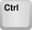+ 1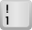on the keyboard, select custom on the left side and type “ddd” for the abbreviation or “dddd” for the full name of the weekday.
• Option 2: Copy and paste this formula: =CHOOSE(WEEKDAY(NOW()),”Sunday”,”Monday”,”Tuesday”,”Wednesday”,”Thursday”,”Friday”,”Saturday”)
• You want to know how much time has past since your last update? Let’s say, cell A1 contains a fixed date:
=NOW()-A1
You want to convert this into…
• Whole days: =ROUNDDOWN((NOW()-A1),0)
• Hours: =(NOW()-A1)*24
• Minutes: =(NOW()-A1)*24*60
• Seconds: =(NOW()-A1)*24*60*60
• You want to know how old you are? Let’s assume, your birthday is given in cell A1.
• The easiest way (but unfortunately not very exact due to leap years with one more day): =(NOW()-A1)/365  or =(NOW()-A1)/365.25  . The second option is a little bit more exact though but also not 100%.
• Better: =YEARFRAC(A2,NOW()) .

## Time stamp: How to insert a static date and time which doesn’t update

The problem with the NOW formula: It changes every time Excel calculates. So you can consider it always up-to-date. But sometimes you just want to get a time stamp. Here are 4 methods of how to enter a fixed date and time:

1. Enter it manually: E.g. just type 3/27/2017 10:47 AM and press enter.
2. Use keyboard shortcuts:
1. Press Ctrl+ ;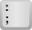on the keyboard for the current date.
2. Press Ctrl+ Shift+ ;on the keyboard for the current date.
3. Now you can combine these two keyboard shortcuts: First insert the current date and then the current time.
3. The third method: Enter the NOW() formula, copy it and paste special it as values. Again, step by step:
1. Enter =NOW() into a formula.
2. Go to the cell in which you’ve just entered the NOW formula and copy it by pressing the keys Ctrl+ c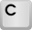.
3. Paste it by pressing Ctrl+ Alt+ von the keyboard. Select “Values” (press v) and press enter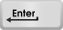.
4. Use the following VBA macro. Therefore, insert a new module and for convenience purpose add a button which starts the macro. For more information please refer to this guide (chapters “How to insert a new VBA module manually” and “How to run your VBA macro”).
``````Sub updateTimeStamp()
ActiveCell = Now()
End Sub``````

## Difference to the TODAY formula

There is another very similar formula in Excel: the formula =TODAY() returns todays date. Let’s see the difference to =NOW():

• =NOW() returns the exact date and time. The underlying number could be something like 42423.9230527778. The number 42423 describes the day whereas the decimals contain the information about the time.
• =TODAY() only returns the whole number 42423.
• Both formulas are volatile. That means they will be calculated each time Excel calculates something – even if there are no changes.Henrik Schiffner is a freelance business consultant and software developer. He lives and works in Hamburg, Germany. Besides being an Excel enthusiast he loves photography and sports.